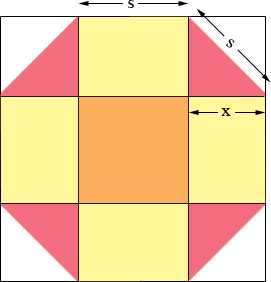SEARCH HOMEMath Central Quandaries & QueriesQuestion from Heidi, a student: Draw an octagon with an area of 18 square centimetersHeidi,

I think of a regular octagon as a square with the 4 corners lopped off.I let the length of the side of the octagon be s cm, chopped it up as in the diagram and introduced the variable x. The octagon is then partitioned into 9 regions

1 square of area s2 cm2
4 rectangles each of area xs cm2
4 triangles

Two triangles can be put together along the hypotenuse to form a square which is x cm by x cm. Thus the total area of the regular octagon is

s2 + 4 xs + 2 x2 = 18 cm2

Each pink triangle is a right triangle with side lengths x, x and s cm. Thus by Pythagoras theorem

x2 + x2 = s2

Solve this equation for s, substitute into the equation for the area and solve for x. Once you have x you can use x2 + x2 = s2 to find s.

Construct a square with side length s + 2x cm. Measure x cm in from each corner and cut off the corners. The resulting regular octagon has an area of 18 square centimeters.

PennyMath Central is supported by the University of Regina and The Pacific Institute for the Mathematical Sciences.